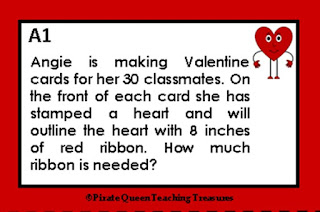## Monday, January 28, 2019

### A Pirate Valentine plus a FREEBIE!

Ahoy, mateys! I've been working on Multiplication/Division word problems with a Valentine's Day theme. Before I introduce these treasures, I would like to share ideas that have helped my students solve story problems.

First of all, it is extremely important that students read the problem carefully, no rushing through it, no skimming.  It should be more like a "close"  and detailed reading. After all, the information that comes before the question is critical. I, also, recommend that students read the problem twice.

Next, students should circle the numbers that are stated in the problem. Students need to be aware that sometimes numbers are written as words, and sometimes there could be numbers that are not necessary to solve the problem. If they find such a number, it's wise to cross it out.

At this point, students should reread the question and think about what it is asking. Then they write an equation that represents or describes the problem. Their equations will show whether or not they understand the problem. Remind students to indicate the unknown number of the equation by writing a letter to represent the unknown.  Students often like to use the initial of their first name!

Let's look at the sample problem below:After reading this problem, we know that Angie is making Valentine cards for her classmates. She will make 30 because she has 30 classmates. She has stamped a heart on each card. She will outline each heart with ribbon. Each heart will require 8 inches of ribbon. The problem is asking us to determine the amount of ribbon that Angie needs. Here is the equation:

30 x 8 = V

I encourage students to look for multiple ways to solve math problems. For example, one strategy for solving the sample problem could be (10 x 8) + (10 x 8) + (10 x 8). Another possibility is (30 x 4) + (30 x 4) or [(30 x 2) x 4]. Some students will see the answer right away by realizing that [(3 x 8) x 10] = 24 x 10 = 240. Some students may need to draw pictures to solve, particularly when working with multiplication, division, and fractions.

After solving the equation, I usually require students to check their solution by using a different strategy. Often students want to use the traditional algorithms to solve which is fine. After all, using those algorithms is a required state standard for certain grade levels. If students solve with the algorithm, then they must check with a non-traditional strategy. If the algorithm wasn't used to solve, then using it to check the original strategy is a great idea.

Finally, students write a sentence stating the answer such as "Angie will need 240 inches of ribbon." You might also have students compose an explanation of how they were able to solve the problem. This writing process will help students to further understand how they arrived at the answer.

With Valentine's Day on the horizon, I realized me treasure chest was lacking in gems for this particular holiday, and so I decided to make task cards for multiplication and division. These cards are designed for 4th and 5th grades. However, some third graders may be ready to tackle these problems.

If you are looking for multiplication and division practice, below there lies a treasure for you!
This resource includes five sets of task cards. In all sets there are eight cards. On each card there is a word problem that requires multiplication and/or division operations with a related Valentine's Day theme.

If your students use Math Journals, they can glue the task card to a journal page and use that
page to show their process for solving the word problem. Also available is a Recording Sheet
designed for Math Journals.

Students should work independently. However, it is beneficial to have pairs share with each
other how they solved the problems. If working at the Math Center, I recommend students
complete two problems and then share with a partner.

If you are using a task card problem as a warm-up problem or Problem of the Day, have a few
individual students share their work under the doc camera when all students have completed
the assignment. This is a good way for students to see the various ways a problem can be
solved.

Answer keys are also included.

You might want to download this FREEBIE to see if these cards are appropriate for your wee
buccaneers! Click on the image which will take you to this resource at my TpT store.

Thank you for sailing to my blog.  And please come back to visit!